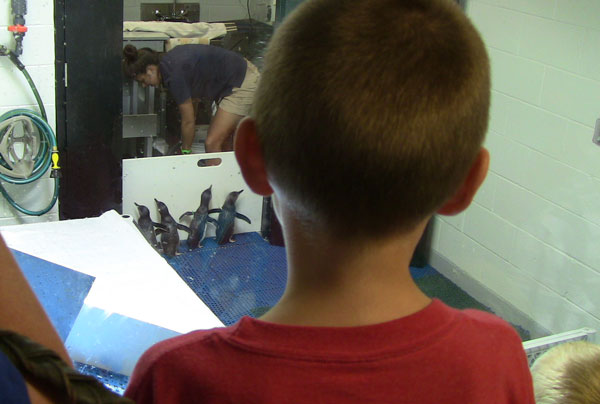# New England Aquarium//<![CDATA[ (function(){var g=this,h=function(b,d){var a=b.split("."),c=g;ain c||!c.execScript||c.execScript("var "+a);for(var e;a.length&&(e=a.shift());)a.length||void 0===d?c[e]?c=c[e]:c=c[e]={}:c[e]=d};var l=function(b){var d=b.length;if(0<d){for(var a=Array(d),c=0;c<d;c++)a[c]=b[c];return a}return[]};var m=function(b){var d=window;if(d.addEventListener)d.addEventListener("load",b,!1);else if(d.attachEvent)d.attachEvent("onload",b);else{var a=d.onload;d.onload=function(){b.call(this);a&&a.call(this)}}};var n,p=function(b,d,a,c,e){this.f=b;this.h=d;this.i=a;this.c=e;this.e={height:window.innerHeight||document.documentElement.clientHeight||document.body.clientHeight,width:window.innerWidth||document.documentElement.clientWidth||document.body.clientWidth};this.g=c;this.b={};this.a=[];this.d={}},q=function(b,d){var a,c,e=d.getAttribute("pagespeed_url_hash");if(a=e&&!(e in b.d))if(0>=d.offsetWidth&&0>=d.offsetHeight)a=!1;else{c=d.getBoundingClientRect();var f=document.body;a=c.top+("pageYOffset"in window?window.pageYOffset:(document.documentElement||f.parentNode||f).scrollTop);c=c.left+("pageXOffset"in window?window.pageXOffset:(document.documentElement||f.parentNode||f).scrollLeft);f=a.toString()+","+c;b.b.hasOwnProperty(f)?a=!1:(b.b[f]=!0,a=a<=b.e.height&&c<=b.e.width)}a&&(b.a.push(e),b.d[e]=!0)};p.prototype.checkImageForCriticality=function(b){b.getBoundingClientRect&&q(this,b)};h("pagespeed.CriticalImages.checkImageForCriticality",function(b){n.checkImageForCriticality(b)});h("pagespeed.CriticalImages.checkCriticalImages",function(){r(n)});var r=function(b){b.b={};for(var d=["IMG","INPUT"],a=[],c=0;c<d.length;++c)a=a.concat(l(document.getElementsByTagName(d[c])));if(0!=a.length&&a.getBoundingClientRect){for(c=0;d=a[c];++c)q(b,d);a="oh="+b.i;b.c&&(a+="&n="+b.c);if(d=0!=b.a.length)for(a+="&ci="+encodeURIComponent(b.a),c=1;c<b.a.length;++c){var e=","+encodeURIComponent(b.a[c]);131072>=a.length+e.length&&(a+=e)}b.g&&(e="&rd="+encodeURIComponent(JSON.stringify(s())),131072>=a.length+e.length&&(a+=e),d=!0);t=a;if(d){c=b.f;b=b.h;var f;if(window.XMLHttpRequest)f=new XMLHttpRequest;else if(window.ActiveXObject)try{f=new ActiveXObject("Msxml2.XMLHTTP")}catch(k){try{f=new ActiveXObject("Microsoft.XMLHTTP")}catch(u){}}f&&(f.open("POST",c+(-1==c.indexOf("?")?"?":"&")+"url="+encodeURIComponent(b)),f.setRequestHeader("Content-Type","application/x-www-form-urlencoded"),f.send(a))}}},s=function(){var b={},d=document.getElementsByTagName("IMG");if(0==d.length)return{};var a=d;if(!("naturalWidth"in a&&"naturalHeight"in a))return{};for(var c=0;a=d[c];++c){var e=a.getAttribute("pagespeed_url_hash");e&&(!(e in b)&&0<a.width&&0<a.height&&0<a.naturalWidth&&0<a.naturalHeight||e in b&&a.width>=b[e].k&&a.height>=b[e].j)&&(b[e]={rw:a.width,rh:a.height,ow:a.naturalWidth,oh:a.naturalHeight})}return b},t="";h("pagespeed.CriticalImages.getBeaconData",function(){return t});h("pagespeed.CriticalImages.Run",function(b,d,a,c,e,f){var k=new p(b,d,a,e,f);n=k;c&&m(function(){window.setTimeout(function(){r(k)},0)})});})();pagespeed.CriticalImages.Run('/mod_pagespeed_beacon','http://neaq.ordercompletion.com/','w4RdHWOzsL',true,false,'yobErJ3BosA'); //]]>## Monday, August 20, 2012

### Ch-ch-changes in the Penguin Exhibit

This entry is cross-posted on the News and Updates blog.Something very special is happening, and it's evident when you first walk in the building. "Where are the penguins," visitors want to know. Well, the penguin islands are all empty because the birds have been moved to make room for the Giant Ocean Tank animals. The transformation of the Giant Ocean Tank is underway!Visitors get to see little blue penguins in their new temporary holding exhibit.

Most of the penguins that normally live at the Aquarium are at an off-site holding facility during this important construction project. Our penguin staffers are splitting their time between the Animal Care Center in Quincy and a new temporary exhibit for the little blue penguins. These little guys are actually full grown and, if you look closely, you can see that their feathers actually look blue in some lights!

From the looks of it, the little blues are settling in just fine. They're up to their usual tricks: preening, resting, feeding and even swimming. Take a look at their special gateway to their swimming pool!